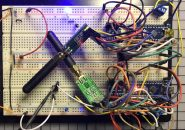## CMUcam5 Pixy视觉相机传感器简介第二部分——创建球平衡梁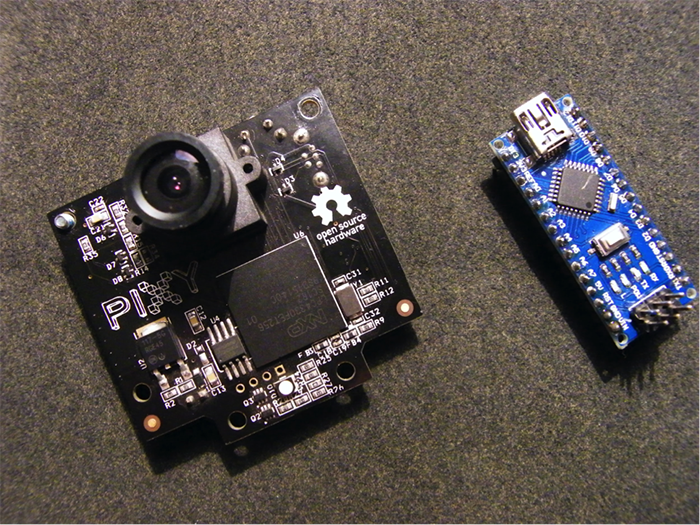• Arduino Uno (您可以使用任何 Arduino)
• CMUcam5 Pixy 相机
• 伺服电机 (S06NF)
• 木片和螺丝
• 数据线（用于相机USB MINI 以及Uno USB B）
• 用于伺服的5V外部电源（！警告！如果您将伺服连接到Arduino通过USB进行供电，您的Arduino将会被烧坏）

• Arduino IDE 1.6.9
• PixyMon 软件 (https://cmucam.org/projects/cmucam5/wiki/Install_PixyMon_on_Windows_Vista_7_8)
• PixyMon 用于 Arduino 的库(https://cmucam.org/projects/cmucam5/wiki/Latest_release)

## Processing的简单介绍Processing是非常有用又灵活的一款软件。它主要用于视觉艺术和科技领域的视觉语言。这款软件具有100多个库，可支持各种项目。它的文档非常齐全，提供了许多使用指南，涵盖了从编程基础到可视化等各种主题。它能够支持所有操作系统（GNU/Linux, Mac OS X, 和 Windows）。该软件的设计几乎和Arduino IDE相同。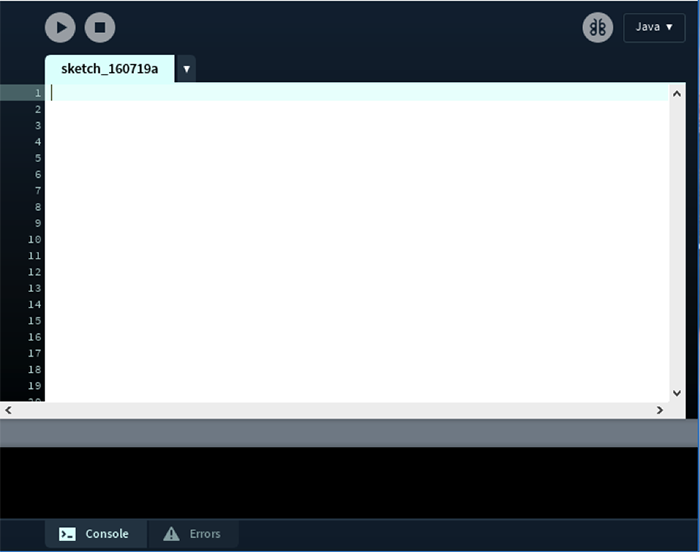## 项目概况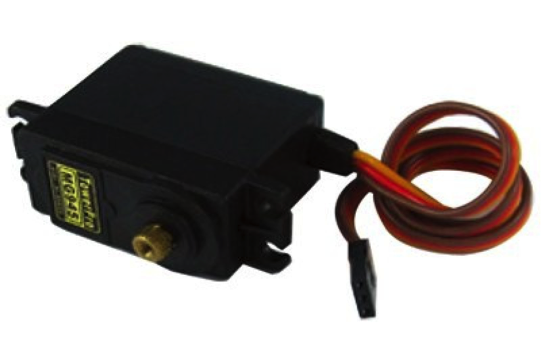## 平衡梁结构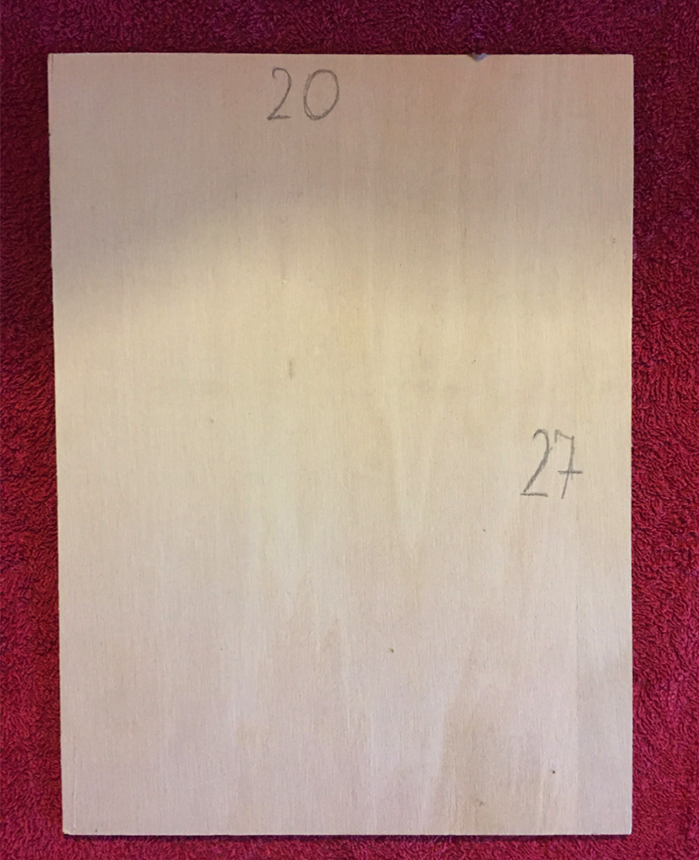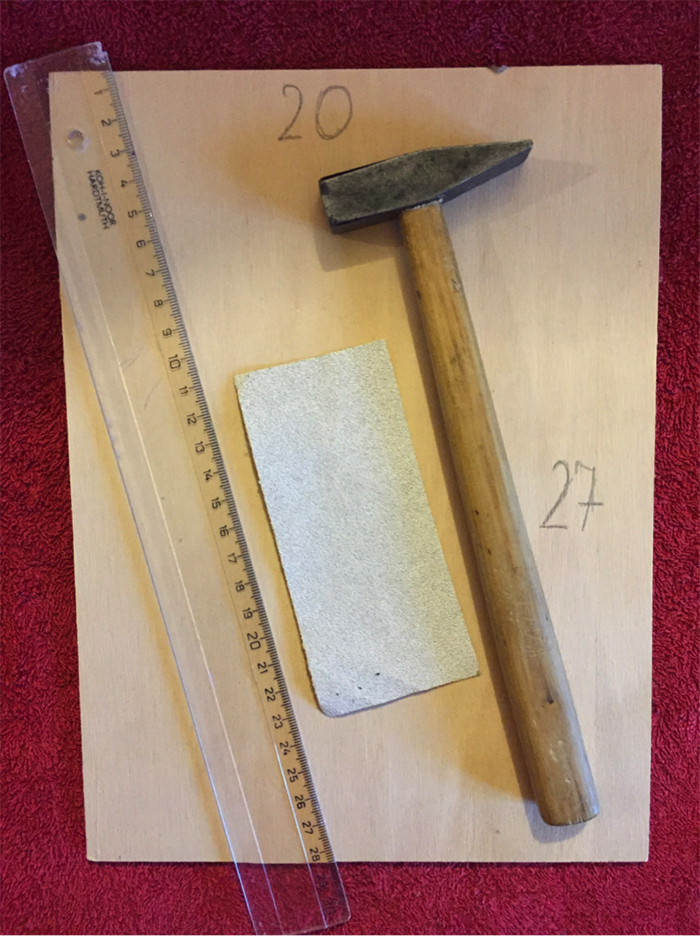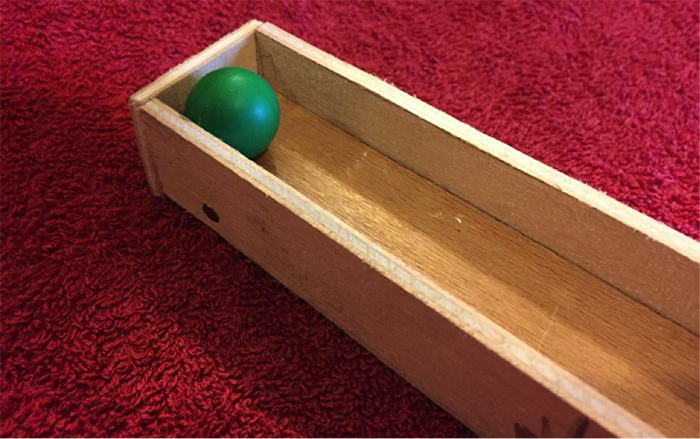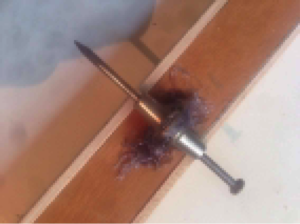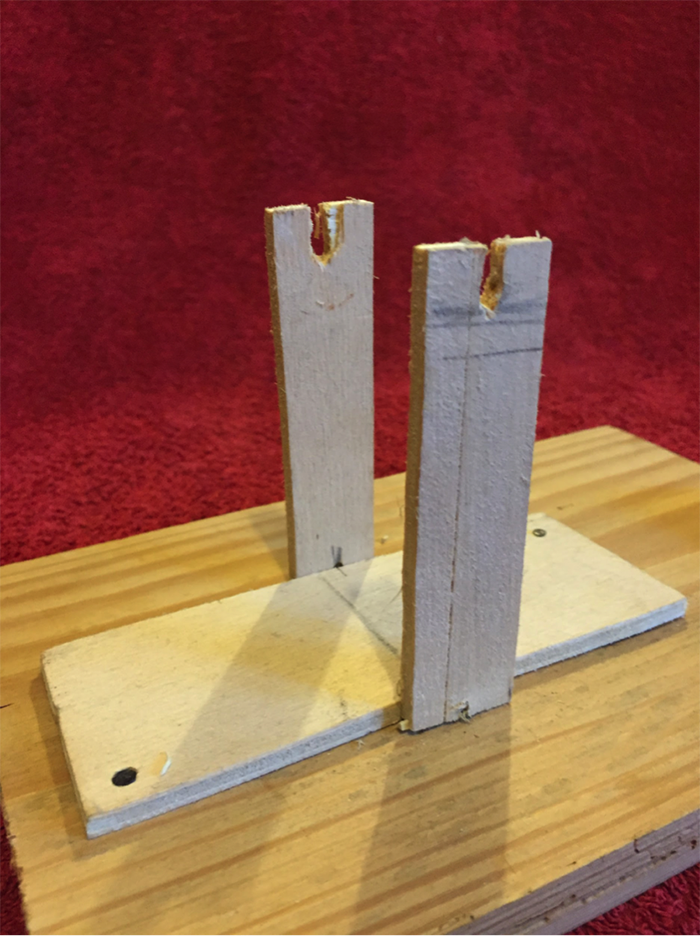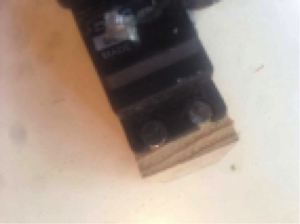## 连接所有部件

！警告！不要忘记连接接地端。如果没有将电源、伺服和Arduino接地端相连接，伺服将会失控！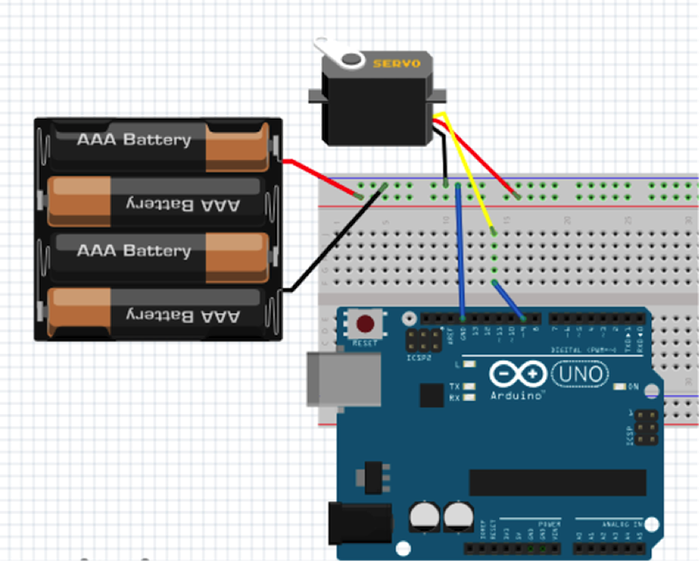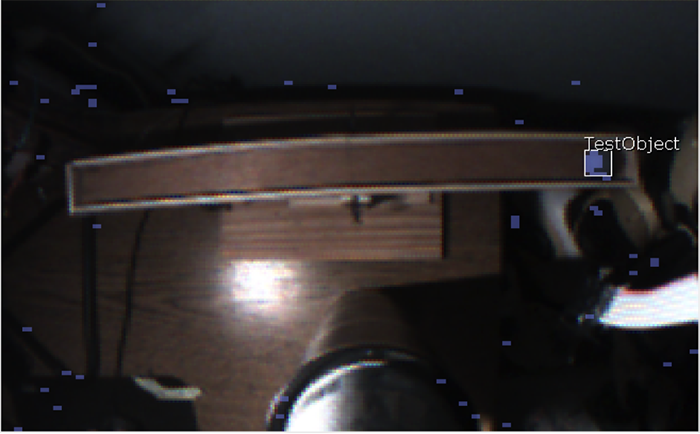``````#include <Servo.h>

uint8_t leveled = 110;     //middle positon for s1 to keep the board leveled

uint8_t far_right = 180;     //far left positon for s1 to keep the board leveled

uint8_t far_left = 0;     //far right positon for s1 to keep the board levele

Servo s;

void setup(){

s.write(leveled);

delay(2000);

s.write(far_right);

delay(2000);

s.write(far_left);

delay(2000);

}

void loop(){

}
``````

### Arduino 代码

``````#include
#include

char val; // Data received from the serial port
int ledPin = 13; // Set the pin to digital I/O 13
void setup() {
pinMode(ledPin, OUTPUT); // Set pin as OUTPUT
Serial.begin(9600); // Start serial communication at 9600 bps
}
void loop() {
if (Serial.available())
{ // If data is available to read,
}
if (val == '1')
{ // If 1 was received
digitalWrite(ledPin, HIGH); // turn the LED on
} else {
digitalWrite(ledPin, LOW); // otherwise turn it off
}
delay(10); // Wait 10 milliseconds for next reading
}
``````

### Processing 代码

``````import processing.serial.*;

Serial myPort;  // Create object from Serial class

void setup()
{
size(200,200); //make our canvas 200 x 200 pixels big
String portName = Serial.list(); //change the 0 to a 1 or 2 etc. to match your port
myPort = new Serial(this, portName, 9600);
}
void draw() {
if (mousePressed == true)
{                       	//if we clicked in the window
myPort.write('1');     	//send a 1
println("1");
} else
{                       	//otherwise
myPort.write('0');      	//send a 0
}
}
``````## 使用Processing编程``````import processing.serial.*;

Serial myPort;        // The serial port
int xPos = 1;         // horizontal position of the graph
float inByte = 0;

void setup () {
// set the window size:
size(400, 300);

// List all the available serial ports
// if using Processing 2.1 or later, use Serial.printArray()
println(Serial.list());

// I know that the first port in the serial list on my mac
// is always my  Arduino, so I open Serial.list().
// Open whatever port is the one you're using.
myPort = new Serial(this, Serial.list(), 9600);

// don't generate a serialEvent() unless you get a newline character:
myPort.bufferUntil('\n');

// set inital background:
background(0);
}
void draw () {
// draw the line:
stroke(127, 34, 255);
line(xPos, height, xPos, height - inByte);

// at the edge of the screen, go back to the beginning:
if (xPos >= width) {
xPos = 0;
background(0);
} else {
// increment the horizontal position:
xPos++;
}
}

void serialEvent (Serial myPort) {
// get the ASCII string:

if (inString != null) {
// trim off any whitespace:
inString = trim(inString);
// convert to an int and map to the screen height:
inByte = float(inString);
println(inByte);
inByte = map(inByte, 0, 1023, 0, height);
}
}
``````

Arduino 代码:

``````#include
#include
#include
#include

//37       	164       	288

uint8_t leveled = 110;    	//middle positon for s1 to keep the board leveled
uint8_t far_right = 180;    	//far left positon for s1 to keep the board leveled
uint8_t far_left = 0;    	//far right positon for s1 to keep the board levele
int current_pos = leveled;
int percentage,var,_percen;

Servo s;
Pixy pixy;

void test_board(){
Serial.write("Starting test");
s.write(leveled);
delay(2000);
s.write(far_right);
delay(2000);
s.write(far_left);
delay(2000);
Serial.write("Finished test, press any key to continue");
s.write(current_pos);
Serial.write("Continued");
}

void setup() {
Serial.begin(9600);
s.attach(9);
pixy.init();
while (!Serial);
//test_board();
s.write(current_pos);
}
void _servo(unsigned char side,int var){
//by the % we get how "hard" we need to wip :D
var = var - 90;
if(side == 'L'){
//Serial.write("LEFT");
//90  180
_percen = 90 + var;
s.write(_percen);
}else{
//Serial.write("RIGHT");
//0  90
_percen = 90 - var;
s.write(_percen);
}
}
void loop() {
static int i = 0;
int j;
uint16_t blocks;
char buf;

// grab blocks!
blocks = pixy.getBlocks();

// If there are detect blocks, print them!
if (blocks)
{
i++;

// do this (print) every 50 frames because printing every
// frame would bog down the Arduino
if (i%1 ==0)
{
//sprintf(buf, "Detected %d:\n", blocks);
//Serial.print(buf);
for (j=0; j<blocks; j++)
{
//sprintf(buf, "  block %d: ", j);
//Serial.print(buf);
//  pixy.blocks[j].print();
uint32_t x_pos= pixy.blocks[j].x;
//Serial.write(x_pos);

percentage = (x_pos - 35) / 2.5;
if(percentage <= 40 && percentage >= 0){
//  Serial.write("LEFT");
var = percentage / 0.4;
_servo('L',var);
}else if(percentage >= 60 && percentage <= 110){
//Serial.write("RIGHT");
var = (percentage - 60) / 0.5;
_servo('R',var);
}else{
//Serial.write("MIDDLE");
}
}
}
}

}
``````

## 代码释义

• 尝试多种算法
• 有多种类型的数学算法可以进行计算。我至少尝试了两到三种，但是最后决定选择该算法。我建议您自己来编写算法，以更好地掌握这种平衡的方法。

• 更好的硬件
• 对于本项目来说，没有什么材料可称得上是完美的，木材就更差得远了。如果我拥有及时可用的资源，那我会选择用金属来建造它，这样整个项目将会更加稳定和精确。

• 变得更快
• 我们如何做到使其更快地恢复平衡？我在这里使用了一个简单的伺服。我们可以将其替换为UART或者AX-12之类的伺服，它们会强大、快速得多。速度也与算法有关。同样，我建议您尝试不同的算法，以找到适用于您的目的的算法。##### Silard Gal

Silard来自塞尔维亚，热爱电子和编程相关的一切事物。他热衷于使用Arduino、Raspberry Pi、 Atmel等创建各种项目，为自己能够成为自主机器人研发团队的一员感到自豪。

### 相关文章

1.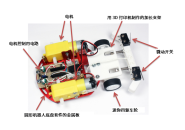#### 在最后一刻停下来！用Arduino和距离传感器制作小鸡赛车！（第3篇•最终篇）

2.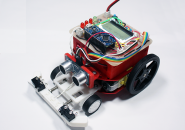#### 在最后一刻停下来！用Arduino和距离传感器制作小鸡赛车！（第2篇）

3.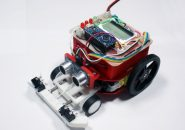#### 在最后一刻停下来！用Arduino和距离传感器制作小鸡赛车！（第1篇）

4.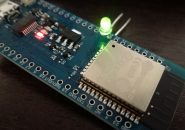#### 通过Arduino使用ESP-WROOM-32（环境搭建和配置-LED闪烁篇）

5.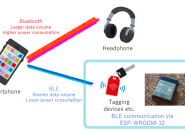#### 通过Arduino使用ESP-WROOM-32（Bluetooth-BLE连接篇）

6.#### 六种可以用于扩展物联网应用的DIY项目

7.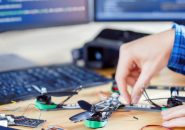#### 使用Arduino的五大DIY无人机项目

8.#### 从电动滑板到无人机：5个DIY项目来提升您的电子技能

9.